## Tip: How to choose a SAS procedure to compute percentiles (quantiles)

Started ‎09-23-2014 by
Modified ‎10-06-2015 by
Views 12,150

# Overview

Computing percentiles (quantiles) is a common task in data mining, and SAS® offers many PROCs for percentile computation or estimation. Those PROCs (in alphabetic order) are: HPBIN, HPSUMMARY, MEANS, STDIZE, SUMMARY and UNIVARIATE. This tip gives you guidelines to help you choose among the available procedures depending on factors such as the size of your data set and available memory. (In this tip, we use the terms “percentiles” and “quantiles” interchangeably.)

But first, let’s consider how percentiles are computed. A straightforward way is to first to sort the data in ascending order and then compute percentiles by counting the observations. Another traditional approach is to use order statistics. However, traditional approaches often fail with large data sets due to lack of memory. Fortunately, the SAS® high-performance procedures (HPBIN and HPSUMMARY) are able to execute in single machine mode and distributed mode. In the distributed mode, several computing nodes participate in the calculations therefore we have access to more memory and enhanced computing capability. Thus, in distributed mode, SAS® high-performance procedures can handle very large data sets. NOTE: Distributed mode requires SAS® High-Performance Server Distributed Mode.

# PROC HPBIN

PROC HPBIN  can compute the 0% (minimum), 1%, 5%, 10%, 25%, 50%, 75%, 90%, 95%, 99%, and 100% (maximum) percentiles of each input variable. To avoid the time-consuming sorting process, the HPBIN procedure uses an iterative projection method to compute percentiles. The HPBIN procedure runs in either single-machine mode or distributed mode. It exploits all the available  cores and concurrent threads, regardless of execution mode. In the following SAS code, a toy data set with one thousand observations and one numeric variable is created for the purpose of demonstration. To compute the percentiles (quantiles), you need use the COMPUTEQUANTILE option in the PROC HPBIN statement.

data test;

call streaminit(2014);

do i=1 to 1000;

x=rand('UNIFORM');

output;

end;

run;

proc hpbin data=test out=hpbin computeQuantile;

var x;

ods output Quantile=Quantile;

run;

The following screenshots show the performance information of PROC HPBIN and the quantiles of the input variable.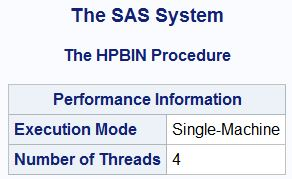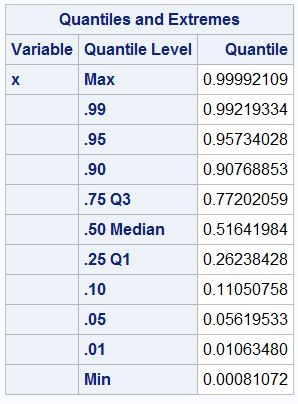To run this example in distributed mode, you need to specify two macro variables for the GRIDHOST= and GRIDINSTALLLOC=options. The NODES= and NTHREADS options specify the number of nodes and number of threads used in PROC HPBIN.

proc hpbin data=test out=hpbin computeQuantile;

var x;

ods output Quantile=Quantile;

run;

# PROC HPSUMMARY

You can use the QNTLDEF= option to specify the mathematical definition that the HPSUMMARY  procedure uses to compute quantiles. HPSUMMARY supports five different definitions of quantiles. The QMETHOD= option specifies the quantile computation method. QMETHOD=OS specifies that PROC HPSUMMARY use order statistics, which can be very memory-intensive. QMETHOD=P2 specifies that PROC HPSUMMARY use the P2 method to approximate the quantile. The P2 is less memory-intensive but reliable estimations of some quantiles (P1, P5, P95, P99) might not be possible for some data sets. In the following example, QNTLDEF=3 and QMETHOD=OS are used.

proc hpsummary data=test QNTLDEF=3 qmethod=OS;

var x;

output out=hpsummary MIN= p1= p5= p10= p25= p50= p75= p90= p95= p99= MAX= / autoname;

run;

proc print data=hpsummary; run;

The following screenshot shows the performance information of PROC HPSUMMARY and the quantiles of the input variable.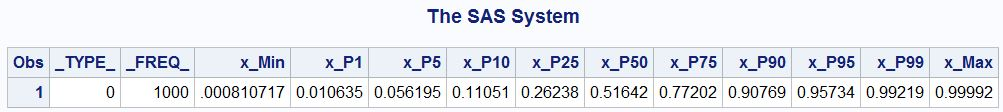To run this example in distributed mode, you need to specify two macro variables for the GRIDHOST= and GRIDINSTALLLOC=options. The NODES= and NTHREADS options specify the number of nodes and number of threads used in PROC HPSUMMARY.

proc hpsummary data=test QNTLDEF=3 qmethod=OS; /* qmethod=P2 for estimation */

var x;

output out=hpsummary MIN= p1= p5= p10= p25= p50= p75= p90= p95= p99= MAX= / autoname;

run;

proc print data=hpsummary; run;

# PROC MEANS and PROC SUMMARY

The syntax of PROC MEANS  and PROC SUMMARY  is consistent with PROC HPSUMMARY (which is the High Performance version of PROC SUMMARY) in quantile computation. In the following example, QNTLDEF=3 and QMETHOD=OS are used.

proc means data=test QNTLDEF=3 qmethod=OS;

var x;

output out=means MIN=p1= p5=p10= p25=p50= p75=p90= p95=p99= MAX= / autoname;

run;

proc print data=means; run;

proc summary data=test QNTLDEF=3 qmethod=OS;

var x;

output out=summary MIN=p1= p5=p10= p25=p50= p75=p90= p95=p99= MAX= / autoname;

run;

proc print data=summary; run;

The following screenshot shows the quantiles of the input variable computed by PROC MEANS and PROC SUMMARY. The results are identical from the two PROCs and therefore only one table is shown here. Please note that there is no performance information table since these two PROCs are not SAS® high-performance procedures.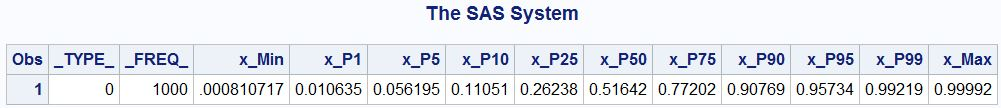# PROC STDIZE

PROC STDIZE  offers two methods for computing quantiles: the order-statistics method and the one-pass method, which is a variant of the P2 method. In the following example, PCTLDEF=3 and PCTLMTD=ORD_STAT are used.

proc stdize data=test outstat=stdize PCTLDEF=3 PCTLMTD=ORD_STAT

pctlpts=(0 1 5 10 25 50 75 90 95 99 100);

var x;

run;

proc print data=stdize;run;

The following screenshot shows the quantiles of the input variable computed by PROC STDIZE. There is no performance information table since PROC STDIZE is not a SAS® high-performance procedure.# PROC UNIVARIATE

PROC UNIVARIATE  automatically computes the 0% (minimum), 1%, 5%, 10%, 25%, 50%, 75%, 90%, 95%, 99%, and 100% (maximum) percentiles of each input variable. Order statistics method is used in percentile computation. It also supports five different definitions of percentiles. In the following example, PCTLDEF=3 is used.

proc univariate data=test PCTLDEF=3;

var x;

ods output Quantiles=Quantiles;

run;

The following screenshot shows the quantiles of the input variable computed by PROC UNIVARIATE. There is no performance information table since PROC STDIZE is not a SAS® high-performance procedure.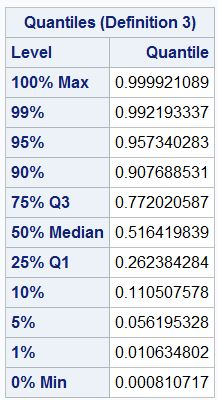# Summary

SAS® offers a number of PROCs for percentile computation and estimation. When the input data set is small, all of them can be used for accurate percentile computation. When the input data set is big, some PROCs (HPSUMMARY, MEANS, SUMMARY, and STDIZE) may use the P2 method for percentile estimation. When the input data set is very big, PROC HPBIN can be used for percentile computation and PROC HPSUMMARY can be used for percentile estimation. The following table gives rules of thumb for choosing among them for quantile computation or estimation. In the following table, "Exact" means the result is accurate and "Estimation" means the result may be an approximation, and "Not Recommended" means the procedure may run out of memory in certain situation therefore it is not recommended. Please note that this table is just a rough summary based on only the number of observation in your data set. The number of variables also impact the memory usage. All of the procedures will eventually run out of memory if the size of the data set is beyond the capability of your computing resources.

HPBIN HPSUMMARY MEANS SUMMARY STDIZE UNIVARIATE
small data set (up to a few millions of observations) Exact Exact Exact Exact Exact Exact
big data set (up to hundreds of millions of observations) Exact Estimation Estimation Estimation Estimation Not Recommended
very big data set (billions of observations) Exact Estimation Not Recommended Not Recommended Not Recommended Not Recommended

References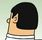Very interesting, crystal clear and comprehensive ! Thanks a lot. This article I wish I'd known long before really fills a gap in (my) SAS knowledge.

When the quantiles to be calculated are equal-sized and not unevenly spaced (the latter being assumed here), as with deciles complete list (P10, P20 ... P80, P90) or even percentiles (P1, P2 ...P98, P99,P100 ), the list of procedures then becomes shorter, it seems - please, correct me if I'm wrong  :

• Proc UNIVARIATE (instruction PCTLPTS)
• Proc STDIZE (id.)
• Proc HPBIN (instruction NUMBIN)
Version history
Last update:
‎10-06-2015 01:34 PM
Updated by:Contributors
Article Labels
Article Tags